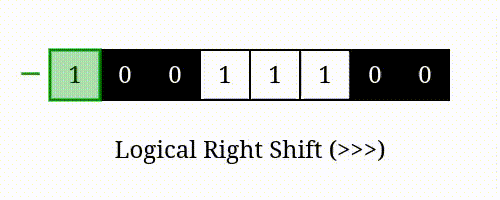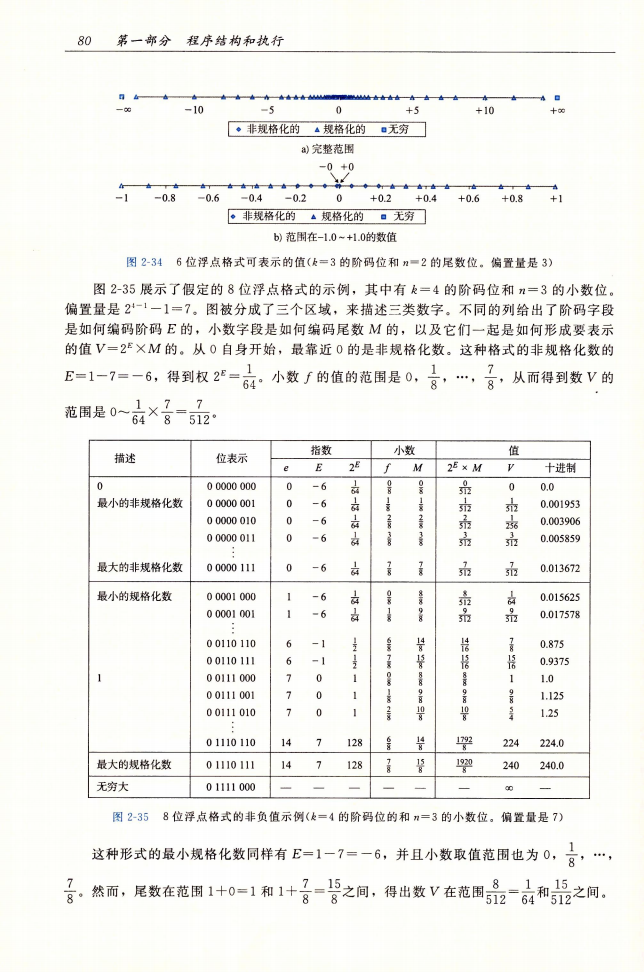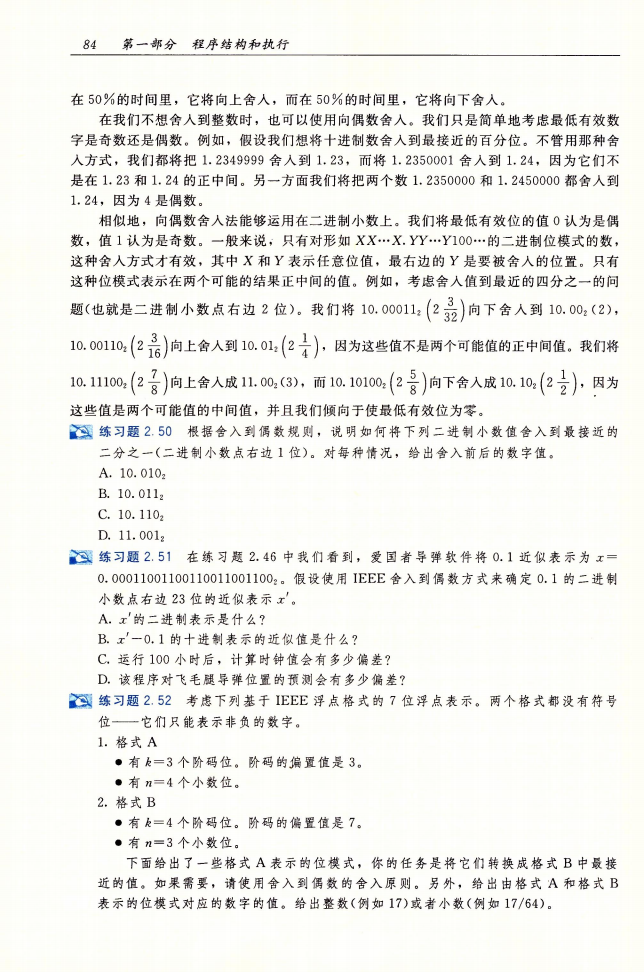23年3月23日，我在公司进行了一次分享会，内容是本文的内容。在分享前，我重新对文章知识点进行了梳理，补充了很多细节。现将补充的细节重新编写到本文中。

# 什么是二进制数？#

• 我们日常使用的是十进制，数字包括0,1,2,3,4,5,6,7,8,9 再往下数，就得向前进一位，变成10，然后从个位数开始继续增加11,12,13…19
• 计算机最底层使用的是二进制，数字包括0和1，再往下数，也是前进一位，变成10。注意，这个10并不是十进制的十，而是十进制的二。

00000
00011
00102
00113
01004
01015
01106
01117
10008
10019
101010
101111
110012
110113
111014
111115

# 二进制如何表示负数？#

## 原码#

3

1 011

### 原码表示负数存在的问题#

1. 0000和1000，都是表示数字0，但是一个是正0，一个是负0。这显然不符合我们对零的理解。
2. 无法进行加减运算：观察以下式子1(0001) + (-3(1011)) = -4(1100)   0001 +1011 -——-   1100

## 补码#

1. 把8往逆时针方向旋转到6（蓝色）这种方式就是进行8-2=6
2. 把8往顺时针方向旋转到6（黄色）这种方式是进行8+10=18，但是时钟只能显示12个数字，所以18-12=6

### 尝试用补码进行运算#

0001

+      x

-——–

0000

X只能表示正数的话，那么这是一个无解的方程。 但是如果我们假设答案存在第五位的1，那么就可以进行运算

0001

+      x

-——–

10000

0010

+      x

-——–

1111

1111-1
00011
1101-3
00113

000000
000111
001022
001133
010044
010155
011066
011177
10008-8
10019-7
101010-6
101111-5
110012-4
110113-3
111014-2
111115-1

### 补码表示的新理解#

1. 想获得某个二进制补码表示的最小的数，只需要让其符号位为1，其他位为0即可。 比如在5位表示数的情况下，最小的数是-16，即10000。
2. 想获取-1，只需要让所有位都为1即可，那么想获得-1，可以直接用0按位取反。

# 二进制数的加法和乘法#

## 二进制数的加法#

• 二进制的加法与十进制的计算规则是一样的，当某一位的数大于1时，往前进一位。
• 对于无符号二进制数和补码二进制数，将数的每一位进行加法运算。
• 在不溢出的情况下，考虑两个4位无符号二进制数的加法：4+6=10：

0100

+0110

-——–

1010

1000

+1000

-——–

10000

12

x 34

-——–

48

36

-——–

408

0011

x 0101

-——–

0011

0011

-——–

001111

# 二进制按位左移和右移#

## 逻辑右移### 算数右移#

• 在右移一位后，左边的符号位被复制之后保留下来。原来是负数的数，现在依旧还是负数
• 对于补码二进制数，算数右移n位，视为除以2的n次方（整除）。如1100(-4) » 1 = 1110(-2)；1100 » 2 = 1111(-1)

## 按位移动位的数量，系统默认帮你取模#

``````int a = 1, b = 32;

print("%d, %d", a<<b, 1<<32);
``````

a « b 的结果是1，是因为运行时会将操作数b对32取模，然后在进行移位操作。

# 布尔代数#

• 按位与

0 & 0 = 0；1 & 0 = 0；0 & 1 = 0；1 & 1 = 1。

• 按位或

0 | 0 = 0；1 | 0 = 1；0 | 1 = 1；1 | 1 = 1。

• 按位非

~1 = 0；~0 = 1。

• 按位异或

0 ^ 0 = 0；1 ^ 0 = 1; 0 ^ 1 = 1; 1 ^ 1 = 0。

1. 异或有一个性质是：a ^ a = 0， (a ^ b) ^ a = b（因为0 ^ b = b）
2. (x | -x) » 31 ，当x为0时依旧为0，当x不为0时，为-1

# C语言unsigned int，会导致问题#

``````
int a = { 1, 2, 3, 4, 5 };

int cnt = 5;
printf("show values\n");
for (int j = 0;j < cnt;j++) {
printf("a[%d]=%d\n", j, a[j]);
}

int i;
printf("start loop\n");
for (i = cnt -2;i >= 0;i--) {
printf("in loop,%u %u\n", i, cnt);
a[i] += a[i+1];
}
printf("end loop\n");

printf("show values\n");
for (int j = 0;j < cnt;j++) {
printf("a[%d]=%d\n", j, a[j]);
}
``````

``````show values
a=1
a=2
a=3
a=4
a=5
start loop
in loop,3 5
in loop,2 5
in loop,1 5
in loop,0 5
end loop
show values
a=15
a=14
a=12
a=9
a=5
``````

``````
int a = { 1, 2, 3, 4, 5 };

int cnt = 5;
printf("show values\n");
for (int j = 0;j < cnt;j++) {
printf("a[%d]=%d\n", j, a[j]);
}

size_t i;
printf("start loop\n");
for (i = cnt -2;i >= 0;i--) {
printf("in loop,%u %u\n", i, cnt);
a[i] += a[i+1];
}
printf("end loop\n");

printf("show values\n");
for (int j = 0;j < cnt;j++) {
printf("a[%d]=%d\n", j, a[j]);
}
``````

``````show values
a=1
a=2
a=3
a=4
a=5
start loop
in loop,3 5
in loop,2 5
in loop,1 5
in loop,0 5
in loop,4294967295 5
in loop,4294967294 5
Segmentation fault (core dumped)
``````

``````
int a = { 1, 2, 3, 4, 5 };

int cnt = 5;
printf("show values\n");
for (int j = 0;j < cnt;j++) {
printf("a[%d]=%d\n", j, a[j]);
}

size_t i;
printf("start loop\n");
for (i = cnt -2;i < cnt;i--) {
printf("in loop,%u %u\n", i, cnt);
a[i] += a[i+1];
}
printf("end loop\n");

printf("show values\n");
for (int j = 0;j < cnt;j++) {
printf("a[%d]=%d\n", j, a[j]);
}
``````

``````show values
a=1
a=2
a=3
a=4
a=5
start loop
in loop,3 5
in loop,2 5
in loop,1 5
in loop,0 5
end loop
show values
a=15
a=14
a=12
a=9
a=5
``````

# 浮点数#

a * 10b

## IEEE754规范下的浮点数#

• s是符号位，0为正数，1为负数
• M是尾数，是一个二进制小数。在规格化的浮点数中，1<=M<2；在非规格化的浮点数中，0<=M<1。
• E是阶码，是一个有符号整数

## 十进制与二进制对比#

• 十进制

• 二进制 公式：(-1)s * M * 2E 要求：
• s 用来表示符号，0正1负
• M 尾数，是二进制小数，规格化浮点数下，1 ≤ M <2
• E 阶码 是一个整数

## 二进制表示#

• 一个单独的符号位s直接编码符号s
• n位的小数字段frac编码尾数M，但编码出来的值也依赖于解码字段的值是否等于0
• k位的阶码字段exp编码阶码E## 尾数M#

IEEE754规定，在计算机内部保存M时，默认这个数的整数部分总是1，所以可以被舍去，只保留后面的xxxxx小数部分。

### 尾数M具体怎么表示？#

M在二进制中存储在frac区域，其读数方式，是假定在frac位部分前已经有一个固定的小数点。

frac为110000…00(2) ，计算 .110000..00(2) 的十进制值为0.75(10),再加上1，为1.75(10)

## 阶码E#

### 阶码E具体怎么表示？#

E在二进制中存在exp区域，其读数方式是将其作为一个无符号二进制数读出数值。

Bias = 2k-1 – 1 , 其中k是exp区域的位数。当k为8时，bias=127

E = exp – bias

### 阶码E的几种情况#

exp部分表示的位，在以下几种不同的情况，表示的数和计算方式略有不同。

1. exp不全为0也不全为1时，浮点数采用之前的读数方式，减去bias得到E。（规格化的数）
2. exp全为0时，这时候的计算E的公式变为E=1-bias，与此同时，计算M的公式也改为M=frac。这么做是为了表示0，和数值非常接近于0的数。（非规格化的数）
3. exp全为1时，当frac部分全为0时，表示无穷大；当frac部分不全为0时，表示“不是一个数”

#### 无穷大#

IEEE754浮点数可以表示无穷大，当exp 的所有位全为1，frac的所有位全为0时即可表示无穷大。

0.111…111* 2-127

## 关于浮点数，非常形象的一张图#

1. 0
2. 非规格化部分
3. 规格化部分
4. 无穷
5. 不是一个正数（NaN）图片来自《深入理解计算机系统》第三版第80页。

## 举个例子计算浮点数#

### 计算规格化的值#

• 尾数M=1+frac
• 阶码E=exp-Bias
• 其中偏置Bias = 2k-1 - 1 ， 注意，这里的k是指exp阶码位的位数 如0.875在8位浮点数（s符号-1位 exp阶码位-4位 frac尾数位-3位）中，如下表可查二进制位表示为：

00110110

(-1)s * M * 2E

s=0

#### 第二部分：尾数M#

frac位=110。我们假设在110前方有一个二进制的小数点，那么frac为 .110

110
0.50.250.125

frac 二进制.110=十进制0.5+0.25=0.75 M = 1 + frac = 1 + 0.75 = 1.75

#### 第三部分：阶码E#

E=exp-Bias

k = 4（因为exp阶码是4位）；

Bias = 2k-1 - 1 = 2 4-1 -1 = 8-1=7

exp = 0110 = 6

E = exp - Bias = 6 - 7 = -1

(-1)s * M * 2E

• s = 0
• M = 1.75
• E = -1

## 计算非规格化的值#

• 尾数M=frac
• 阶码E=1-Bias
• 偏置Bias = 2^（k-1） - 1

00000011

(-1)s * M * 2E

### 第二部分：尾数M#

011
0.50.250.125

frac 二进制.110=十进制0.25+0.125=0.375

M = frac = 0.375

### 第三部分：阶码E#

k = 4（因为exp阶码是4位）；

Bias = 2k-1 - 1 = 2 4-1 -1 = 8-1=7

E = 1-7 = -6

(-1)s * M * 2E 我们有：

• s = 0
• M = 0.375
• E = -6

## 浮点数的“舍入”#图片来自《深入理解计算机系统》第三版第84页。